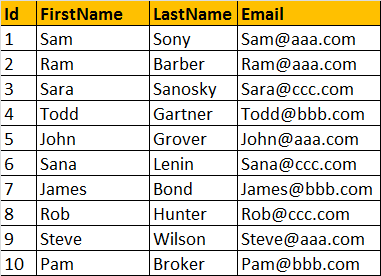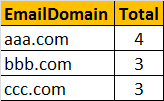HI WELCOME TO SIRING

# LEFT, RIGHT, CHARINDEX and SUBSTRING functions - Part 23

we will learn about the commonly used built-in string functions in SQL server and finally, a real time example of using string functions.

LEFT(Character_Expression, Integer_Expression) - Returns the specified number of characters from the left hand side of the given character expression.

ExampleSelect LEFT('ABCDE', 3)
Output: ABC

RIGHT(Character_Expression, Integer_Expression) - Returns the specified number of characters from the right hand side of the given character expression.

ExampleSelect RIGHT('ABCDE', 3)
Output: CDE

CHARINDEX('Expression_To_Find', 'Expression_To_Search', 'Start_Location') - Returns the starting position of the specified expression in a character string. Start_Location parameter is optional.

Example: In this example, we get the starting position of '@' character in the email string 'sara@aaa.com'.
Select CHARINDEX('@','sara@aaa.com',1)
Output: 5

SUBSTRING('Expression', 'Start', 'Length') - As the name, suggests, this function returns substring (part of the string), from the given expression. You specify the starting location using the 'start' parameter and the number of characters in the substring using 'Length' parameter. All the 3 parameters are mandatory.

Example: Display just the domain part of the given email 'John@bbb.com'.
Select SUBSTRING('John@bbb.com',6, 7)
Output: bbb.com

In the above example, we have hardcoded the starting position and the length parameters. Instead of hardcoding we can dynamically retrieve them using CHARINDEX() and LEN() string functions as shown below.

Example:
Select SUBSTRING('John@bbb.com',(CHARINDEX('@''John@bbb.com') + 1), (LEN('John@bbb.com') - CHARINDEX('@','John@bbb.com')))
Output: bbb.com

Real time example, where we can use LEN(), CHARINDEX() and SUBSTRING() functions. Let us assume we have table as shown below.Write a query to find out total number of emails, by domain. The result of the query should be as shown below.Query
Select SUBSTRING(Email, CHARINDEX('@', Email) + 1,
LEN(Email) - CHARINDEX('@', Email)) as EmailDomain,
COUNT(Email) as Total
from tblEmployee
Group By SUBSTRING(Email, CHARINDEX('@', Email) + 1,
LEN(Email) - CHARINDEX('@', Email))